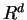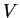Next: Voronoi Diagram and Delaunay Up: Convex Polyhedron Previous: Is there any efficient   Contents

## Is there any efficient algorithm to compute the volume of a convex polytope in?

It is known that computing the volume of a-polytope (or H-polytope) is #P-hard, see [DF88] and [Kha93]. There are theoretically efficient randomized algorithms to approximate the volume of a convex body [LS93] but no implementation seems to be available.

There is a comparative study [BEF00] of various volume computation algorithms for convex polytopes. It indicates that there is no single algorithm that works well for many different types of polytopes. For ``near'' simple polytopes, triangulation-based algorithms are more efficient. For ``near'' simplicial polytopes, sign-decomposition-based algorithms are better. See the paper for the justification of these claims.

Komei Fukuda 2004-08-26